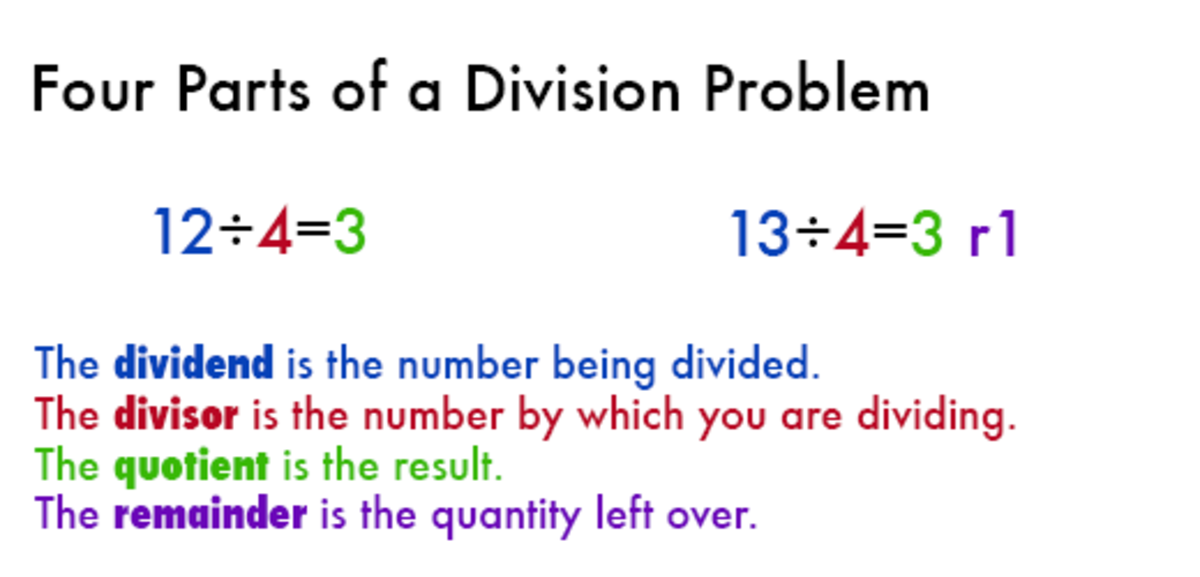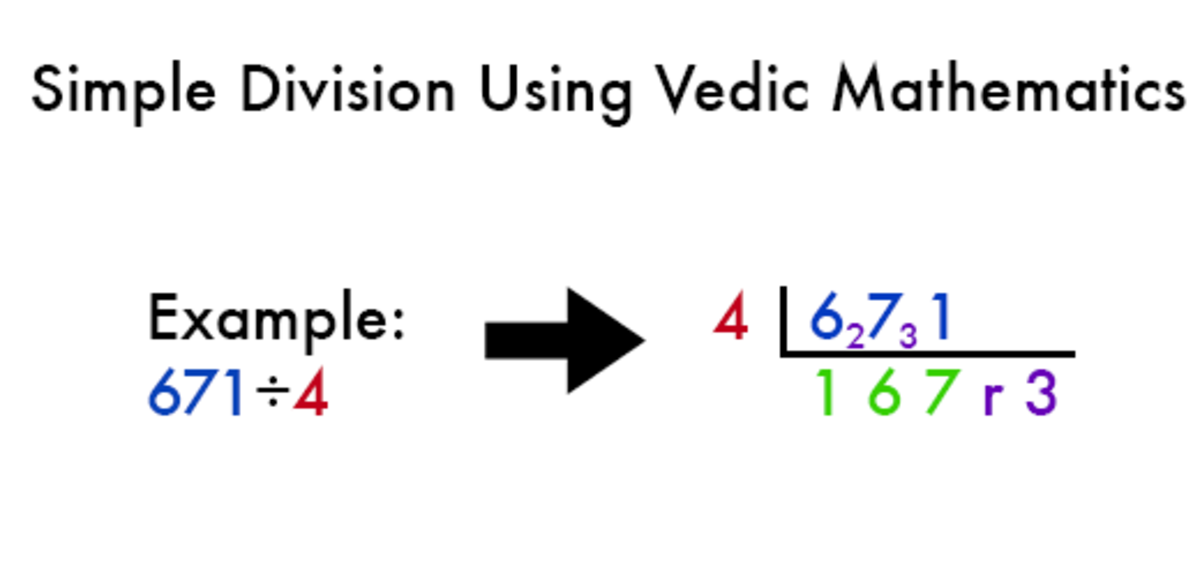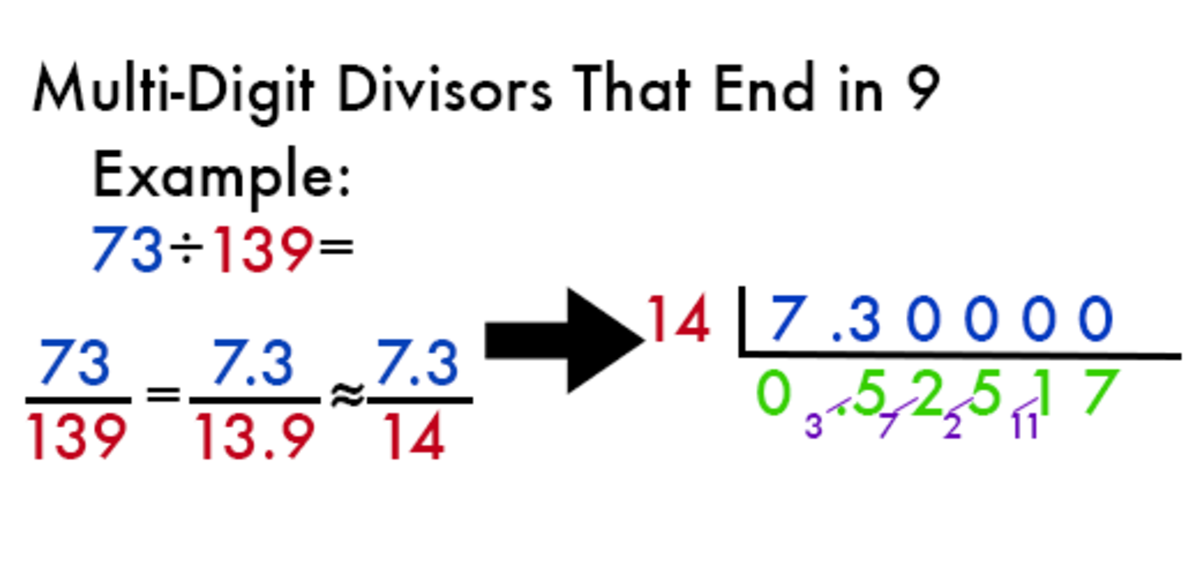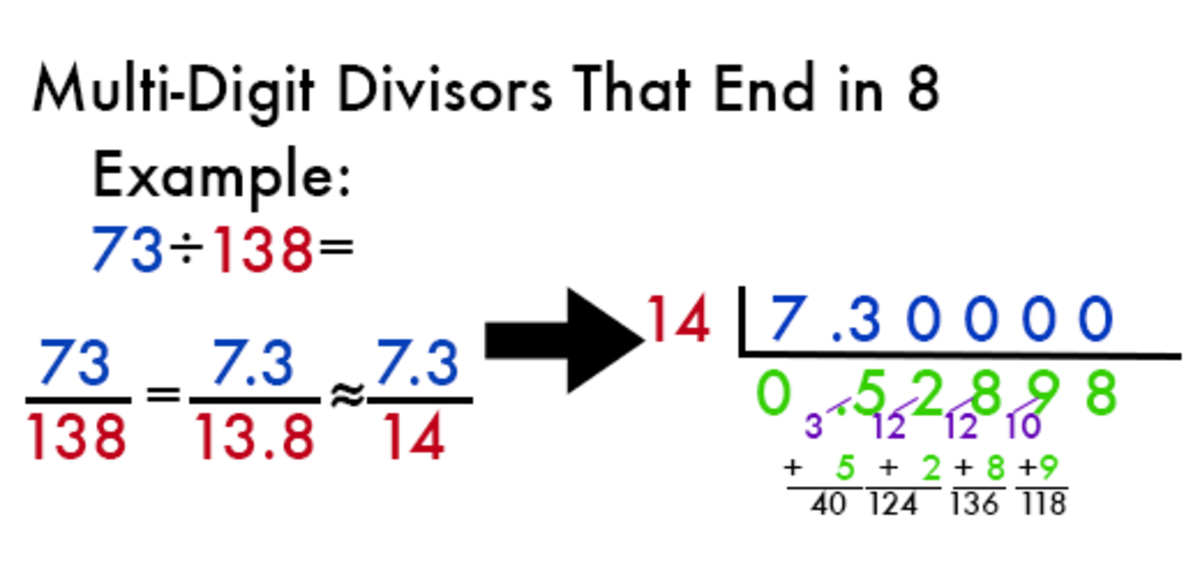# Divide Numbers Easily Using Vedic Mathematics

I am a B.E Graduate from Nagpur University and have a great interest in subjects like Vedic Mathematics, Numerology and Astrology.

## What Is Vedic Mathematics?

Vedic mathematics is a technique for solving algebra quickly and simply. It was invented by Bharati Krishna Tirthaji, who published a book with the same title in 1965. Tirhaji was a famous Hindu cleric, and he claimed to have discovered the technique in ancient sacred Hindu texts.

Whether or not he really did is debatable; what is not is that the math checks out. Whether you want to be able to effortlessly split a check, impress your friends, or learn a different way to quickly divide numbers, this tried-and-true method can be learned within minutes.The four vocabulary words you will need to know in order to follow these division directions.

## Key Terms

Above are the four vocabulary words that you will need to know in order to divide. If you are having a hard time keeping them straight, consider the following:

• A dividend is the number you have beforehand.
• A divisor is the number doing the dividing, just like an advisor is the one doing the advising.
• The only number anyone ever wants to quote is the answer or quotient.
• What remains after you finish dividing is the remainder.An example of simple Vedic division.

## Simple Vedic Division

Set it up:

Write the divisor before the dividend, then box off the left and bottom sides of the dividend in order to keep it visually separate.

Steps to divide:

• 4 into 6 = 1 remainder 2. Write the 2 next to the following digit, 7, making it 27.
• 4 into 27 = 6 remainder 3. Write the 3 next to the following digit, 1, making it 31.
• 4 into 31 = 7 remainder 3.
• The answer is 167 remainder 3.

## Vedic Division with Decimals

What if you don't want a remainder? In that case, you can add a decimal point and 0s behind the dividend and continue the process.

• Write the remainder, 3, next to the following digit, 0, making it 30.
• 4 into 30 = 7 remainder 2. Write the 2 next to the following digit, 0, making it 20.
• 4 into 20 = 5 remainder 0. Since the remainder is 0, you have already passed the decimal point, and if there are no more values greater than 0, you have completed the problem.
• The answer is 167.75.
Scroll to Continue

## Read More From Owlcation

In the example above, you can see that once you have passed the decimal point and no values greater than zero remain to the right, you are finished as soon as there is no remainder.

## You Try

Solve question two from the practice problems to the nearest thousandth place.

## How Do You Use Vedic Division When the Divisor Is More Than One Digit?

That's simple enough, but how do you use Vedic division when the divisor has more than one digit? The technique depends on what digit the divisor ends in. See the example below to learn how to divide with a divisor that ends in 9.Vedic division example with a divisor that ends in 9.

## Multi-Digit Divisor Ending in 9 Example

Set it up:

Division can also be expressed as a fraction; here, 73 divided by 139 is the same thing as 73 over 139. Divide both the numerator and denominator of the fraction (the top and bottom number) by 10 so that the 9 is behind the decimal point. Then round the denominator (the bottom number) up—in this case, round up 13.9 to 14.

Then, like before, write the divisor before the dividend, then box off the left and bottom sides of the dividend in order to keep it visually separate.

Steps to divide (we'll round to the nearest ten-thousandth):

• 14 does not go into 7, so write 0 followed by a decimal point.
• 14 into 73 = 5 remainder 3. Make a note of the remainder, 3, in front of the 5, making it 35.
• 14 into 35 = 2 remainder 7. Make a note of the remainder, 7, in front of the 2, making it 72.
• 14 into 72 = 5 remainder 2. Make a note of the remainder, 2, in front of the 5, making it 25.
• 14 into 25 = 1 remainder 11. Make a note of the remainder, 11 in front of the 1, making it 111.
• 14 into 111 = 7 remainder 13.
• The answer is 0.52517, which rounds to 0.5252.Vedic division example with a divisor that ends in 8.

## Multi-Digit Divisor Ending in 8 Example

Set it up:

Follow the same set up as the previous problem. Here, 73 divided by 138 is the same thing as 73 over 138. Divide both the numerator and denominator of the fraction (the top and bottom number) by 10 so that the 8 is behind the decimal point. Then round the denominator (the bottom number) up—in this case, round up 13.8 to 14.

Then, like before, write the divisor before the dividend, then box off the left and bottom sides of the dividend in order to keep it visually separate.

Steps to divide (we'll round to the nearest ten-thousandth):

• 14 does not go into 7, so write 0 followed by a decimal point.
• 14 into 73 = 5 remainder 3. Make a note of the remainder, 3, in front of the 5, making it 35. Then add the quotient, 5, to 35 to get 40.
• 14 into 40 = 2 remainder 12. Make a note of the remainder, 12, in front of the 2, making it 122. Then add the quotient, 2, to 122 to get 124.
• 14 into 124 = 8 remainder 12. Make a note of the remainder, 12, in front of the 8, making it 128. Then add the quotient, 8, to 128 to get 136.
• 14 into 136 = 9 remainder 10. Make a note of the remainder, 10 in front of the 9, making it 109. Then add the quotient, 9, to 109 to get 118.
• 14 into 118 = 8 remainder 6.
• The answer is 0.52898, which rounds to 0.5290.

## How Do You Use Vedic Division When the Divisor Ends In a Digit Other Than 8 or 9?

The only difference between dividing by a divisor that ends in 8 and one that ends in any other digit is that you will add the quotient a different number of times. For divisors that end in 8, you add the quotient once in each step; for divisors that end in 7, you will add it twice, and so on. See the chart below for how many times you will add it for different end numerals.

## Vedic Division with Multi-Digit Divisors

If last digit is a 0, after the set up, you can carry on with simple Vedic division.

The End Numeral of the DivisorSet Up (Always the Same)First Part of Each Step (Always the Same)How Many Times You Add the Quotient

9

Set up the division problem as a fraction. Divide the top and bottom by 10 and round the denominator up.

Find the quotient and remainder. Write down the quotient, then write the remainder before it.

Add the quotient 0 times.

8

Set up the division problem as a fraction. Divide the top and bottom by 10 and round the denominator up.

Find the quotient and remainder. Write down the quotient, then write the remainder before it.

Add the quotient 1 time.

7

Set up the division problem as a fraction. Divide the top and bottom by 10 and round the denominator up.

Find the quotient and remainder. Write down the quotient, then write the remainder before it.

Add the quotient 2 times.

6

Set up the division problem as a fraction. Divide the top and bottom by 10 and round the denominator up.

Find the quotient and remainder. Write down the quotient, then write the remainder before it.

Add the quotient 3 times.

5

Set up the division problem as a fraction. Divide the top and bottom by 10 and round the denominator up.

Find the quotient and remainder. Write down the quotient, then write the remainder before it.

Add the quotient 4 times.

4

Set up the division problem as a fraction. Divide the top and bottom by 10 and round the denominator up.

Find the quotient and remainder. Write down the quotient, then write the remainder before it.

Add the quotient 5 times.

3

Set up the division problem as a fraction. Divide the top and bottom by 10 and round the denominator up.

Find the quotient and remainder. Write down the quotient, then write the remainder before it.

Add the quotient 6 times.

2

Set up the division problem as a fraction. Divide the top and bottom by 10 and round the denominator up.

Find the quotient and remainder. Write down the quotient, then write the remainder before it.

Add the quotient 7 times.

1

Set up the division problem as a fraction. Divide the top and bottom by 10 and round the denominator up.

Find the quotient and remainder. Write down the quotient, then write the remainder before it.

Add the quotient 8 times.

Mathfluencer on June 26, 2019:

Hey sarat, you can get only approximate result and it is .904 :)

sarat on March 18, 2019:

Hi, i am not getting correct answer for 88/97 .can you help to solve the problem in getting the correct answer

amerah kaaied on February 09, 2019:

i am in 4th grad and i wood like some tricks for divishon .

Harshit Setia on October 03, 2018:

It is wrong formula and wewant the formula of 12

rishav on August 03, 2018:

Good

Himal on December 18, 2017:

3850÷ 3.1416

Shweta on November 28, 2017:

How to divide 12064 by 12 using this formula in 3 ways

Salman Haidar Laskar on August 16, 2017:

I was very good &interesting

Jitendra on July 08, 2017:

How we can divide 1 by any 5 digit number quickly

mickey goel on June 06, 2017:

not liked so much

jaspreet on November 06, 2016:

That's great ..

H on October 16, 2016:

Yaaaaa................ It is helpfull

durgaprasad on May 03, 2016:

very nice

dsfhskajdf on July 12, 2015:

very inter.. i have never seen it before in my life ♂☺☺♪♫☼

dex on July 11, 2015:

This isnt really vedic math, this is the same as long division

Rishav on May 06, 2015:

It really helped thanks very much.

TheGuy on January 13, 2015:

What you have explained is not vedic methods. If you do not know, please do not post such things and insult vedic knowledge. These are more or less western methods.

Kiran on July 07, 2014:

How to do the same on three digits/three digits (ex: 248/179) and three digits/four digits (ex: 248/3578). Please help

sanket on July 01, 2014:

Thnx.. It helped

hinal on February 04, 2014:

I thank you very much ...grateful to you if you do this with examples

------------- on September 03, 2013:

......................................

Jatish on August 09, 2013:

I thank you very very very..........................................much. But will you explain in detail how to divide when the denominator is 7,6,5,4,3,2,1.

I would be very...........................grateful to you if you do this with examples

vivekkumar on May 17, 2013:

great trick ........just keep doing the good work

ramees.p on June 21, 2012:

thanx its amazing

Shehzeen on May 21, 2012:

Its nice n amazing

wafa on May 09, 2012:

very nice and amazing

rahul on May 05, 2012:

Hey thanx mate it works lyk magic . . .but these tricks aren't hlping me in some kind of problems like 21/22 or 73/84 nd many others, would please hlp me out wid these kind of problems :-)

Hary on May 01, 2012:

Great technique and thanks a lot.

shiv paliwal on August 04, 2011:

really supreb.............

BJT on July 24, 2011:

Nice technique, keep publish these type of methods, I will publish in my facebook wall with ur name.

jastine kaye gaitano on July 16, 2011:

i really like it it help me very much thanks :)

Michael Greenlaw on May 31, 2011:

This blog is full of mistakes.

Answers are not correct.

• 75/168=7.5/16.8=75./17= 0. 5 3 9 5 6 8 –Answer

5 13 7 9 11 --Remainder

This a problem with divisors ending in 9 not 8.

Annswer is incorrect

varsha on April 16, 2011:

Finally!!!a good vedic maths site!!!Liked dis method a lot!!!

shehra on February 03, 2011:

woooow that's amazing

shah alam on January 13, 2011:

very nice method

Cameron on September 29, 2010:

Glad to know the tricks. but not enough to fill the thirst of my zeal to know.

rik on May 17, 2010:

Is there an extension to this, say to divide a number like 1349 by 19 that is similar to the dividibg by 9?

Jyoti on December 05, 2009:

Veri nice informative blog!